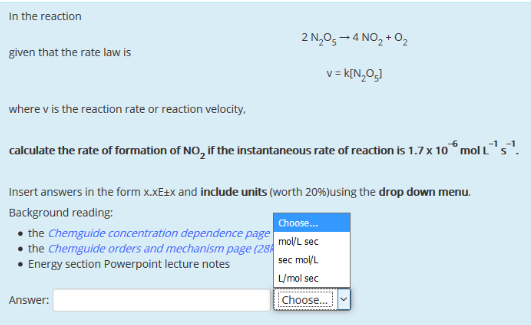# Problem: In the reaction 2N2O5 → 4NO2 + O2 given that the rate law is v = k[N 2O5] where v is the reaction rate or reaction velocity, calculate the rate of formation of NO 2 if the instantaneous rate of reaction is 1.7 x 10-6 mol L-1 s-1.

###### FREE Expert Solution
98% (198 ratings)###### Problem Details

In the reaction

2N2O5 → 4NO2 + O2

given that the rate law is v = k[N 2O5

where v is the reaction rate or reaction velocity, calculate the rate of formation of NO 2 if the instantaneous rate of reaction is 1.7 x 10-6 mol L-1 s-1